# Changing Acceleration in an Electromagnetics question

• Kosta1234
In summary, the problem involves a charge -q falling towards a plane with a positive charge density and then towards a charged sphere. The question is to determine the time it takes for the charge to reach the plane and the center of the sphere. The solution involves using kinematics or energy conservation, but since the force is not constant, the differential equation of motion must be derived and solved. The potential at the point where the charge hits the plane is assumed to be zero.

## Homework Statement

Hello. I'm stuck on some question, will glad if you can give me a little hint.
The problem is this: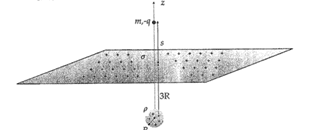A charge -q is located at the distance 's' above a plane, which charged in a positive charge density for area unit σ > 0 .
There is a sphere with the radius R charged on a density for volume p > 0, located 3R under the plane.

the charge -q is "falling" towards the plane, touches it (It tells to ignore the friction) and keep "falling" towards the sphere.

In which time does it touches the plane, and when it reaches the center of the sphere?

## Homework Equations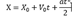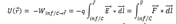## The Attempt at a Solution

Well at first I thought this question is very easy.
To know at how much time the charged "hitted" the plane I can use kinematics or the conservation of energy.

By kinematics method I can find the acceleration using the Electric Field of the plane ( E = σ/(2*eps))
eps * is the electric constant.
and the Electric field of the sphere that is:
0 when r < R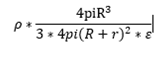when r > R
* I've a mistake - it's without R - only 4pi*r^2
but then I've noticed that the force that given by F = qE is not constant and therefore the acceleration is not constant.

How can I know when it will hit if the acceleration is a function of the distance to the sphere?

thank you.

#### Attachments

Last edited by a moderator:
Kosta1234 said:
How can I know when it will hit if the acceleration is a function of the distance to the sphere?
You will need to derive and solve the differential equation of its motion.
Taking s to be the height at time t, can you write an expression for ##\ddot s##?

You say "falling" but I assume gravity is not to be considered.

Yes. I've meant that is attracting to it.

Well I tried to solve it in two ways:
The first one is using Energy Conservation:

To find the velocity in the moment it hits the plane I used the following equation: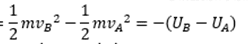when using Va = 0 and Ua = 0.

and I found Ub using:The Electric field is the summary of the two fields created by the plane and by the sphere.

and I hope I got the answer right.
When I used the 2nd Newton's law and Kinetics I've got the same answer.

Hope I got this right, the only problem is wutth Ua = 0. Can I say that the potential in this point is zero?

thank you.

#### Attachments

Kosta1234 said:
Yes. I've meant that is attracting to it.

Well I tried to solve it in two ways:
The first one is using Energy Conservation:

To find the velocity in the moment it hits the plane I used the following equation:
View attachment 229616
when using Va = 0 and Ua = 0.

and I found Ub using: View attachment 229617

The Electric field is the summary of the two fields created by the plane and by the sphere.

and I hope I got the answer right.
When I used the 2nd Newton's law and Kinetics I've got the same answer.

Hope I got this right, the only problem is wutth Ua = 0. Can I say that the potential in this point is zero?

thank you.
I do not see how the methods you describe gave you the time taken.